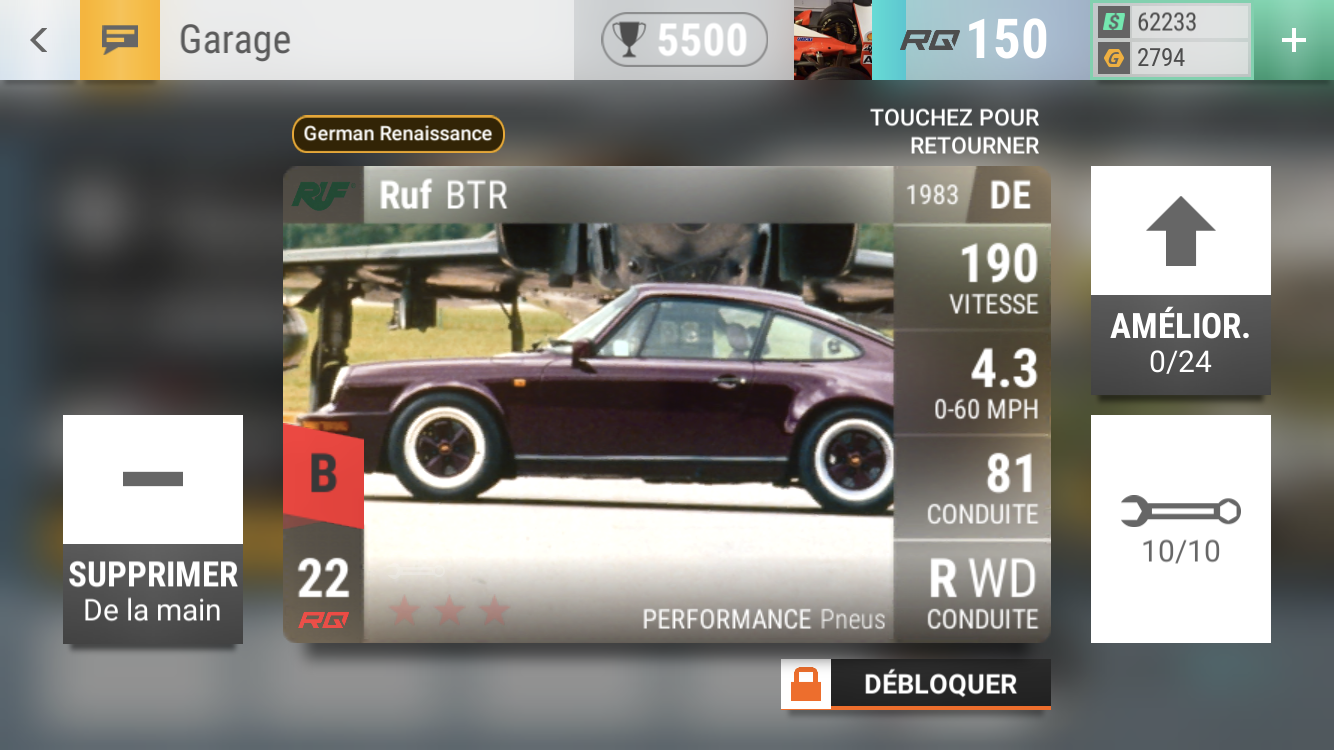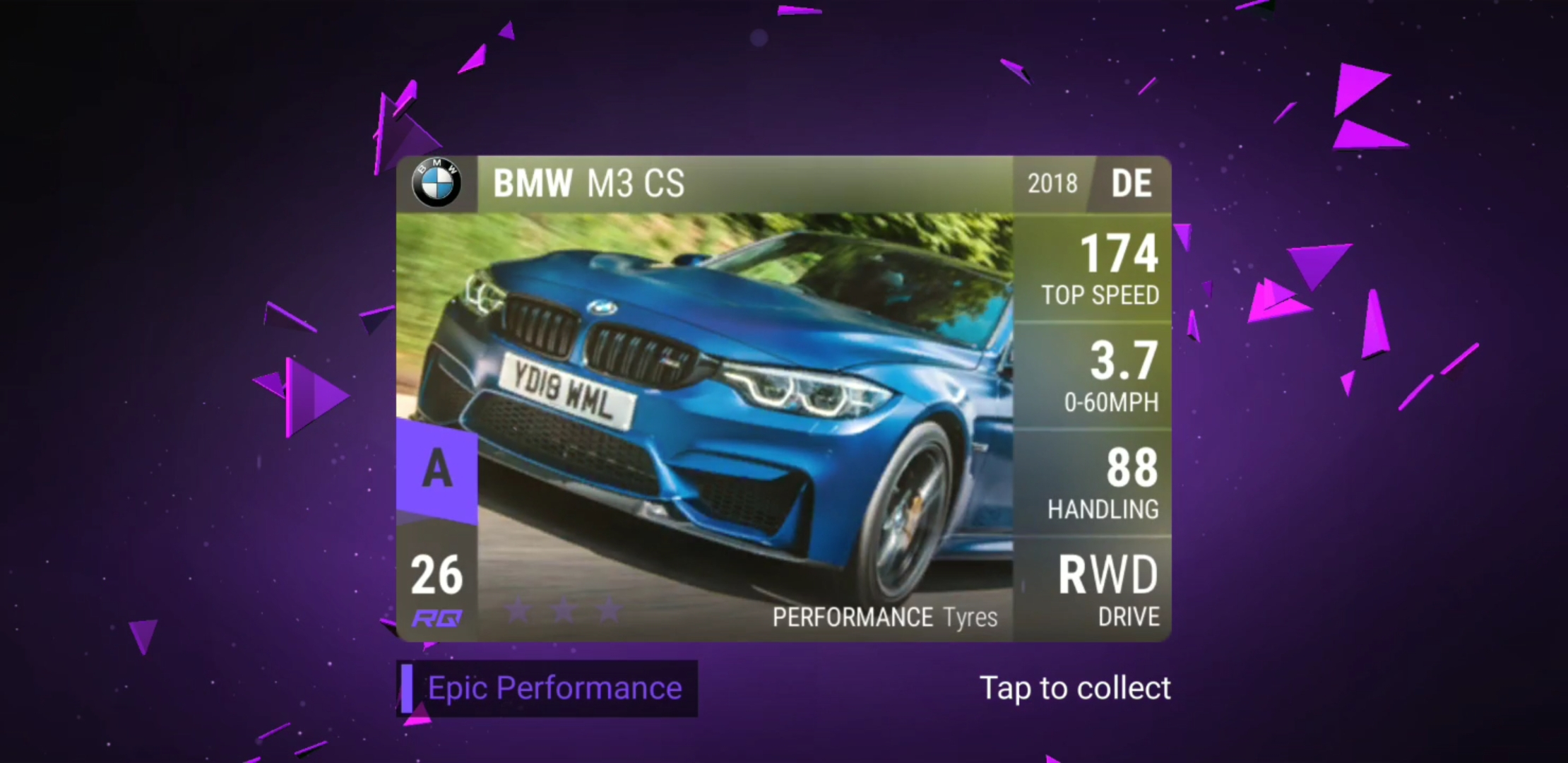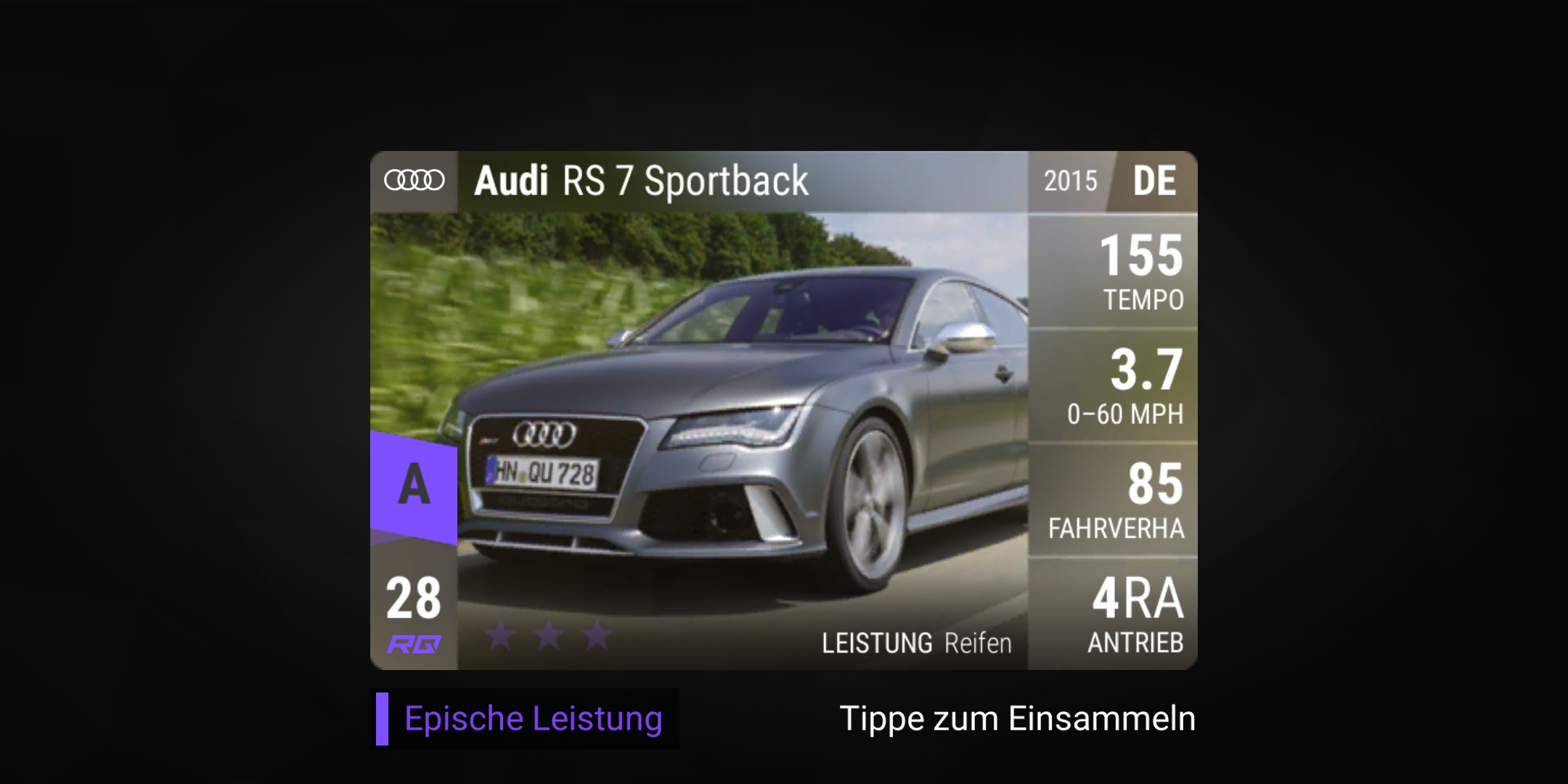# Decent pulls... All my S and A recent pulls

•mra 71.4
• S3XY said:mra 71.4
Soooo it's basically a potato
• S3XY said:mra 71.4
Soooo it's basically a potato
Yeah. rq27 might be accurate to describe how bad it is.
The only use of this car I can imagine is to scare opponents away, but it needs to be accompanied by some other Legendaries.
• S3XY said:mra 71.4
I m sorry to ask this maybe a dumb question, but how do you calculate the MRA?
• Anzatoro said:
S3XY said:mra 71.4
I m sorry to ask this maybe a dumb question, but how do you calculate the MRA?
100 * (acc0_60 / (acc0_100 - acc0_60)) so if your car's 0-60 is 3.8 and the 0-100 is 9.2 you can calculate it with this formula 100*(3.8/(9.2-3.8))=70.37 which means your car's MRA is 70.37
• DING097 said:
Anzatoro said:
S3XY said:mra 71.4
I m sorry to ask this maybe a dumb question, but how do you calculate the MRA?
100 * (acc0_60 / (acc0_100 - acc0_60)) so if your car's 0-60 is 3.8 and the 0-100 is 9.2 you can calculate it with this formula 100*(3.8/(9.2-3.8))=70.37 which means your car's MRA is 70.37
If this formula is reliable then the MRA for the RUF BTR should be around 107. Which is insane for a B-Class car with these kind of stats:• edited June 2019
lg29 said:
DING097 said:
Anzatoro said:
S3XY said:mra 71.4
I m sorry to ask this maybe a dumb question, but how do you calculate the MRA?
100 * (acc0_60 / (acc0_100 - acc0_60)) so if your car's 0-60 is 3.8 and the 0-100 is 9.2 you can calculate it with this formula 100*(3.8/(9.2-3.8))=70.37 which means your car's MRA is 70.37
If this formula is reliable then the MRA for the RUF BTR should be around 107. Which is insane for a B-Class car with these kind of stats:You might be doing it in kmh? I got to 81.13 doing it with mph
• TD42792 said:
lg29 said:
DING097 said:
Anzatoro said:
S3XY said:mra 71.4
I m sorry to ask this maybe a dumb question, but how do you calculate the MRA?
100 * (acc0_60 / (acc0_100 - acc0_60)) so if your car's 0-60 is 3.8 and the 0-100 is 9.2 you can calculate it with this formula 100*(3.8/(9.2-3.8))=70.37 which means your car's MRA is 70.37
If this formula is reliable then the MRA for the RUF BTR should be around 107. Which is insane for a B-Class car with these kind of stats:You might be doing it in kmh? I got to 81.13 doing it with mph

How can he do it in kmh? The acceleration on the card is in mph and also the track is 0-100mph so he cant get a kmh outcome cause all the variables are in mph.
• edited June 2019
Anzatoro said:
TD42792 said:
lg29 said:
DING097 said:
Anzatoro said:
S3XY said:mra 71.4
I m sorry to ask this maybe a dumb question, but how do you calculate the MRA?
100 * (acc0_60 / (acc0_100 - acc0_60)) so if your car's 0-60 is 3.8 and the 0-100 is 9.2 you can calculate it with this formula 100*(3.8/(9.2-3.8))=70.37 which means your car's MRA is 70.37
If this formula is reliable then the MRA for the RUF BTR should be around 107. Which is insane for a B-Class car with these kind of stats:You might be doing it in kmh? I got to 81.13 doing it with mph

How can he do it in kmh? The acceleration on the card is in mph and also the track is 0-100mph so he cant get a kmh outcome cause all the variables are in mph.
I dont know, that’s the only explanation I could think of, maybe he converted it!?
• TD42792 said:
Anzatoro said:
TD42792 said:
lg29 said:
DING097 said:
Anzatoro said:
S3XY said:mra 71.4
I m sorry to ask this maybe a dumb question, but how do you calculate the MRA?
100 * (acc0_60 / (acc0_100 - acc0_60)) so if your car's 0-60 is 3.8 and the 0-100 is 9.2 you can calculate it with this formula 100*(3.8/(9.2-3.8))=70.37 which means your car's MRA is 70.37
If this formula is reliable then the MRA for the RUF BTR should be around 107. Which is insane for a B-Class car with these kind of stats:You might be doing it in kmh? I got to 81.13 doing it with mph

How can he do it in kmh? The acceleration on the card is in mph and also the track is 0-100mph so he cant get a kmh outcome cause all the variables are in mph.
I dont know, that’s the only explanation I could think of, maybe he converted it!?
So if it’s 0-60 is 3.1 and it’s 6.0 to 100 is it's MRA 106.89? Maths was never my strong point.
• LittleEnosBurdette said

So if it’s 0-60 is 3.1 and it’s 6.0 to 100 is it's MRA 106.89? Maths was never my strong point.
Yes
• Got it then. 👍🏻
• What is the Twizy’s MRA? Zero?
Or infinity?
• TD42792 said:
Anzatoro said:
TD42792 said:
lg29 said:
DING097 said:
Anzatoro said:
S3XY said:mra 71.4
I m sorry to ask this maybe a dumb question, but how do you calculate the MRA?
100 * (acc0_60 / (acc0_100 - acc0_60)) so if your car's 0-60 is 3.8 and the 0-100 is 9.2 you can calculate it with this formula 100*(3.8/(9.2-3.8))=70.37 which means your car's MRA is 70.37
If this formula is reliable then the MRA for the RUF BTR should be around 107. Which is insane for a B-Class car with these kind of stats:You might be doing it in kmh? I got to 81.13 doing it with mph

How can he do it in kmh? The acceleration on the card is in mph and also the track is 0-100mph so he cant get a kmh outcome cause all the variables are in mph.
I dont know, that’s the only explanation I could think of, maybe he converted it!?
So if it’s 0-60 is 3.1 and it’s 6.0 to 100 is it's MRA 106.89? Maths was never my strong point.
..you're not talking about the BTR anymore, right? It can't possibly make the 0-100 in 6s, even when maxed, can it?
• TD42792 said:
Anzatoro said:
TD42792 said:
lg29 said:
DING097 said:
Anzatoro said:
S3XY said:mra 71.4
I m sorry to ask this maybe a dumb question, but how do you calculate the MRA?
100 * (acc0_60 / (acc0_100 - acc0_60)) so if your car's 0-60 is 3.8 and the 0-100 is 9.2 you can calculate it with this formula 100*(3.8/(9.2-3.8))=70.37 which means your car's MRA is 70.37
If this formula is reliable then the MRA for the RUF BTR should be around 107. Which is insane for a B-Class car with these kind of stats:You might be doing it in kmh? I got to 81.13 doing it with mph

How can he do it in kmh? The acceleration on the card is in mph and also the track is 0-100mph so he cant get a kmh outcome cause all the variables are in mph.
I dont know, that’s the only explanation I could think of, maybe he converted it!?
So if it’s 0-60 is 3.1 and it’s 6.0 to 100 is it's MRA 106.89? Maths was never my strong point.
..you're not talking about the BTR anymore, right? It can't possibly make the 0-100 in 6s, even when maxed, can it?
Sorry no I wasn’t, was just checking how it was done.

• edited June 2019
DING097 said:
Anzatoro said:
S3XY said:mra 71.4
I m sorry to ask this maybe a dumb question, but how do you calculate the MRA?
100 * (acc0_60 / (acc0_100 - acc0_60)) so if your car's 0-60 is 3.8 and the 0-100 is 9.2 you can calculate it with this formula 100*(3.8/(9.2-3.8))=70.37 which means your car's MRA is 70.37

Oh oh i just found maybe a flaw in this formula?

How can you multiply and divide decimal numbers with non-decimal time numbers (seconds in particular)? When you multiply or divide the outcome you get is always decimal.

I really dont get it, so the MRA value is just a vague number that shows the power of the car from 60-100mph? Cause its sure not seconds.

Because when you divide lets say 500 with 6.50seconds the outcome is what? If you do this on a calculator it will take 6.50 as SIX POINT FIVE which is 6 and a half. But this is not the case cause 6.50 is 6 seconds and 50 tenths of second...
• Sorry, I may have misunderstood, but 6.50 seconds is 6 and a half.

5 tenths of a second is .5, which is half, right?
• RWare said:
Sorry, I may have misunderstood, but 6.50 seconds is 6 and a half.

5 tenths of a second is .5, which is half, right?
Ohhh you are absolutely right, it is indeed because its a tenth!
I was totally confused because time is not measured in decimals from minutes and up, but it is measured in decimals on seconds and below.

So you actually can multiply 100*6.3 seconds or divide 500/6.2.
• Anzatoro said:
RWare said:
Sorry, I may have misunderstood, but 6.50 seconds is 6 and a half.

5 tenths of a second is .5, which is half, right?
Ohhh you are absolutely right, it is indeed because its a tenth!
I was totally confused because time is not measured in decimals from minutes and up, but it is measured in decimals on seconds and below.

So you actually can multiply 100*6.3 seconds or divide 500/6.2.
Using this definition mra is a unitless value
• Not a bad duplicate legendary, better than my other duplicate the SLS electricNice, never pulled any GT.
My only duplicate Legendary? Merc SLS....  Ha ha ha ha ha... Sob
• edited June 2019
Not a bad duplicate legendary, better than my other duplicate the SLS electricNice, never pulled any GT.
My only duplicate Legendary? Merc SLS....  Ha ha ha ha ha... Sob
The SLS electric was my first duplicate, got some use out of it in a merc event a while back
• binner said:Timely pull from my Austin challenge cf
Very nice. I’ll just settle for my amazing rq19 🤨
•Would have been happier 2 weeks ago...
Why? Only to get disappointed a week after?
• edited June 2019
binner said:Timely pull from my Austin challenge cf
What Austin CF , am I missing something?

Edit never mind, I've not been paying attention 😂